•5星
135.91MB qq_34351067 2021-04-16 14:11:28
•5星
1.64MB weixin_39589455 2021-02-25 13:45:29
•5星
4.01MB weixin_45739362 2020-11-19 13:47:32
•5星
8.39MB xipengbozai 2021-04-02 08:52:15
•5星
30KB weixin_42325129 2021-06-14 23:11:58
•5星
7KB weixin_40663377 2021-09-04 17:31:39
•5星
3.95MB weixin_42696271 2021-09-10 15:15:58
•5星
14KB weixin_42696333 2021-09-10 17:36:28
•5星
251KB weixin_42696333 2021-09-10 21:01:43
•5星
198KB weixin_48549810 2021-01-06 14:45:40
• C语言实现图的搜索算法示例 图的常用算法c语言

本文实例讲述了C语言实现图的搜索算法。分享给大家供大家参考，具体如下：在游戏中，常常遇到路径规划问题，用到图的相关算法，我们以简单图来学习。图通常有两种表示方式，矩阵和邻接表。矩阵表示简单，运算快，但...

本文实例讲述了C语言实现图的搜索算法。分享给大家供大家参考，具体如下：

在游戏中，常常遇到路径规划问题，用到图的相关算法，我们以简单图来学习。

图通常有两种表示方式，矩阵和邻接表。矩阵表示简单，运算快，但当矩阵是稀疏矩阵的时候就存在空间浪费的问题，并且效率也会下降，而邻接表节约空间，并且由于边是连续访问，时间效率也比较高。在本文中，我们将以邻接表来表示图。

#include

#include

using namespace std;

struct SE{

int vIndex;

int tag;

SE* next;

};

struct SMap{

SE* pE;

int nnode;

};

void visit(SE *se){

printf("%d\n", se->vIndex);

}

SMap* create_map(int matrix[], int n){

SMap* pMap = new SMap();

pMap->nnode = n;

pMap->pE = new SE[n];

memset(pMap->pE, 0, n*sizeof(SE));

for (int i = 0; i

pMap->pE[i].vIndex = i;

pMap->pE[i].tag = 0;

SE* p = &pMap->pE[i];

for (int j = 0; j

if (matrix[i][j] != 0){

p->next = new SE();

p->next->vIndex = j;

p->next->tag = 0;

p->next->next = NULL;

p = p->next;

}

}

}

return pMap;

}

int BFS(SMap* pMap, int n){

queue q;

for (int i = 0; i < n; i++){

if (pMap->pE[i].tag == 0){

q.push(&pMap->pE[i]);

while (!q.empty()){

SE *se = q.front();

q.pop();

if (pMap->pE[se->vIndex].tag == 1){

continue;

}

visit(se);

pMap->pE[se->vIndex].tag = 1;

SE * p = se;

while (p->next){

p = p->next;

if (pMap->pE[p->vIndex].tag == 0){

q.push(p);

}

}

}

}

}

return 0;

}

int DFS(SMap* pMap, int n){

stack s;

for (int i = 0; i < n; i++){

if (pMap->pE[i].tag == 0){

s.push(&pMap->pE[i]);

while (!s.empty()){

SE *se = s.top();

s.pop();

if (pMap->pE[se->vIndex].tag == 1){

continue;

}

visit(se);

pMap->pE[se->vIndex].tag = 1;

SE * p = &pMap->pE[se->vIndex];

stack tmp;

while (p->next){

p = p->next;

if (pMap->pE[p->vIndex].tag == 0){

tmp.push(p);

}

}

while (!tmp.empty()){

s.push(tmp.top());

tmp.pop();

}

}

}

}

return 0;

}

int main(){

int map = {

{ 0, 1, 0, 1, 0, 0 },

{ 1, 0, 1, 1, 1, 0 },

{ 0, 1, 0, 1, 0, 0 },

{ 1, 1, 1, 0, 1, 0 },

{ 0, 1, 0, 1, 0, 1 },

{ 0, 0, 0, 0, 1, 0 }

};

SMap* smap = create_map(map, 6);

// BFS(smap, 6);

DFS(smap, 6);

return 0;

}

希望本文所述对大家C语言程序设计有所帮助。

展开全文weixin_36104484 2021-05-20 13:19:41
• 启发式搜索算法 算法 机器学习

算法步骤：给出初始温度，初始解，退火系数等参数。在温度没有达到降到最终设定的温度时，进行退火过程即进行循环迭代。在该温度下，产生新解，并计算目标函数值。如果目标函数值更优，接受新解。否则，会以一定...

模拟退火：
算法步骤：给出初始温度，初始解，退火系数等参数。（1）在温度没有达到降到最终设定的温度时，进行退火过程即进行循环迭代。（2）在该温度下，产生新解，并计算目标函数值。如果目标函数值更优，接受新解。否则，会以一定的概率接受错误解。该过程完成，即完成一次退火。算法的核心，在与接受概率的设定。
反应在代码上：外循环控制执行次数（进行退火）。（2）内循环搜索最优解。

遗传算法：遗传算法本质上，是一种高效的启发式搜索算法。
算法步骤：（1）产生种群，在此，相当于产生染色体，本质上是对问题的解进行编码。常见的编码方案有：二进制编码，浮点编码等。
（2）计算适应度：适应度的评价由适应度函数（一般和目标函数有关），计算适应度的过程需要解码。
（3）选择：常用方法有：轮盘赌选择，最佳保留选择等。选择的目的，淘汰适应度低的个体，换句话说将劣解舍弃。
（4）交叉：交叉的目的是产生，新的解。这也体现了搜索算法的特点。交叉操作有：单点交叉，两点交叉等。
（5）变异：变异也能够产生新的解，加入变异操作，可以增大解空间，扩大搜索解的范围。变异方法有：均匀变异，非均匀变异等。
经过上述一轮操作后，就产生了一组解。若未达到最大迭代次数，则重复迭代。

import numpy as np
import matplotlib.pyplot as plt
from matplotlib import cm
from mpl_toolkits.mplot3d import Axes3D

DNA_SIZE = 24
POP_SIZE = 200
CROSSOVER_RATE = 0.8
MUTATION_RATE = 0.005
N_GENERATIONS = 50
X_BOUND = [-3, 3]
Y_BOUND = [-3, 3]

def F(x, y):
return 3*(1-x)**2*np.exp(-(x**2)-(y+1)**2)- 10*(x/5 - x**3 - y**5)*np.exp(-x**2-y**2)- 1/3**np.exp(-(x+1)**2 - y**2)

def plot_3d(ax):
X = np.linspace(*X_BOUND, 100)
Y = np.linspace(*Y_BOUND, 100)
X,Y = np.meshgrid(X, Y)
Z = F(X, Y)
ax.plot_surface(X,Y,Z,rstride=1,cstride=1,cmap=cm.coolwarm)
ax.set_zlim(-10,10)
ax.set_xlabel('x')
ax.set_ylabel('y')
ax.set_zlabel('z')
plt.pause(3)
plt.show()

def get_fitness(pop):
x,y = translateDNA(pop)
pred = F(x, y)
return (pred - np.min(pred)) #减去最小的适应度是为了防止适应度出现负数，通过这一步fitness的范围为[0, np.max(pred)-np.min(pred)]

def translateDNA(pop): #pop表示种群矩阵，一行表示一个二进制编码表示的DNA，矩阵的行数为种群数目
x_pop = pop[:,1::2]#奇数列表示X,从索引列1开始，加入了步长2
y_pop = pop[:,::2] #偶数列表示y,从索引列1开始，加入了步长2

#pop:(POP_SIZE,DNA_SIZE)*(DNA_SIZE,1) --> (POP_SIZE,1)
x = x_pop.dot(2**np.arange(DNA_SIZE)[::-1])/float(2**DNA_SIZE-1)*(X_BOUND-X_BOUND)+X_BOUND
y = y_pop.dot(2**np.arange(DNA_SIZE)[::-1])/float(2**DNA_SIZE-1)*(Y_BOUND-Y_BOUND)+Y_BOUND
return x,y

def crossover_and_mutation(pop, CROSSOVER_RATE = 0.8):
new_pop = []
for father in pop:		#遍历种群中的每一个个体，将该个体作为父亲
child = father		#孩子先得到父亲的全部基因（这里我把一串二进制串的那些0，1称为基因）
if np.random.rand() < CROSSOVER_RATE:			#产生子代时不是必然发生交叉，而是以一定的概率发生交叉
mother = pop[np.random.randint(POP_SIZE)]	#再种群中选择另一个个体，并将该个体作为母亲
cross_points = np.random.randint(low=0, high=DNA_SIZE*2)	#随机产生交叉的点
child[cross_points:] = mother[cross_points:]		#孩子得到位于交叉点后的母亲的基因
mutation(child)	#每个后代有一定的机率发生变异
new_pop.append(child)

return new_pop

def mutation(child, MUTATION_RATE=0.003):
if np.random.rand() < MUTATION_RATE: 				#以MUTATION_RATE的概率进行变异
mutate_point = np.random.randint(0, DNA_SIZE*2)	#随机产生一个实数，代表要变异基因的位置
child[mutate_point] = child[mutate_point]^1 	#将变异点的二进制为反转

def select(pop, fitness):    # nature selection wrt pop's fitness
idx = np.random.choice(np.arange(POP_SIZE), size=POP_SIZE, replace=True,
p=(fitness)/(fitness.sum()) )
return pop[idx]

def print_info(pop):
fitness = get_fitness(pop)
max_fitness_index = np.argmax(fitness)
print("max_fitness:", fitness[max_fitness_index])
x,y = translateDNA(pop)
print("最优的基因型：", pop[max_fitness_index])
print("(x, y):", (x[max_fitness_index], y[max_fitness_index]))

if __name__ == "__main__":
fig = plt.figure()
ax = Axes3D(fig)
plt.ion()#将画图模式改为交互模式，程序遇到plt.show不会暂停，而是继续执行
plot_3d(ax)

pop = np.random.randint(2, size=(POP_SIZE, DNA_SIZE*2)) #matrix (POP_SIZE, DNA_SIZE)
for _ in range(N_GENERATIONS):#迭代N代
x,y = translateDNA(pop)
if 'sca' in locals():
sca.remove()
sca = ax.scatter(x, y, F(x,y), c='black', marker='o')
plt.show()
plt.pause(0.1)
pop = np.array(crossover_and_mutation(pop, CROSSOVER_RATE))
#F_values = F(translateDNA(pop), translateDNA(pop))#x, y --> Z matrix
fitness = get_fitness(pop)
pop = select(pop, fitness) #选择生成新的种群
print_info(pop)
plt.ioff()
plot_3d(ax)

粒子群算法：作为启发式搜索算法，和遗传算法在一定程度上，具有相似性，只不过产生新解的方式不同。
算法流程：（1）产生群体的位置和速度。位置：即当前解。速度：更新解的方式。
（2）计算适应度函数，评估解的质量。这点和遗传算法是类似的。
（3）对于每一个粒子，更新它的全局最优位置。当前解更优，用当前解更新该例子的全局最优解。
（4）更新全体最优解，用所有粒子中最优的解来更新全局最优解。
（5）更新粒子的位置和速度，即产生新解，以及下次的更新方式。
（6）算法迭代。

展开全文Pbw_666 2021-08-24 21:57:55
• C++ 常用算法(STL) 算法 数据结构 c++

亦可参考：C++ STL常用算法（排序、合并、搜索和分区） 标准库定义了一组泛型算法：因为它们实现共同的操作，所以称之为“算法”;而“泛型”指的是它们可以操作在多种容器类型上，不但可作用于标准库类型，还可用在...

标准库定义了一组泛型算法：因为它们实现共同的操作，所以称之为“算法”;而“泛型”指的是它们可以操作在多种容器类型上，不但可作用于标准库类型，还可用在内置数组类型，甚至其他类型的序列上大多数算法是通过遍历由两个迭代器标记的一段元素来实现其功能使用泛型算法必须包含头文件算法。：

#include <algorithm>

标准库还定义了一组泛化的算术算法（generalized numeric algorithm），其命名习惯与泛型算法相同。使用这些算法则必须包数字头文件：

#include <numeric>

区别带一个函数参数的算法版本：大部分算法会提供比较或测试函数取代操作符使用的版本，此版本在名字中加了_if后缀。

区别是否实现复制的算法版本：很多算法将重新排列的元素写回其输入范围标准库提供了复制版本，此版本的算法在名字中添加了_copy后缀。

迭代器实参类型

通常泛型算法都是在标记容器（或其他序列）内的元素范围的迭代器上操作的。标记范围的两个实参类型必须精确匹配，它们必须指向同一个容器中的元素（或者超出容器末端的下一位置），并且如果两者不相等，则第一个迭代器通过不断地自增，必须可以到达第二个迭代器对于带有两对迭代器参数的算法，如find_first_of：每对迭代器中，两个实参的类型必须精确匹配，但不要求两对之间的类型匹配。特别是，元素可存储在不同类型序列中，只要这两序列的元素可以比较即可。

一、只读算法

查找算法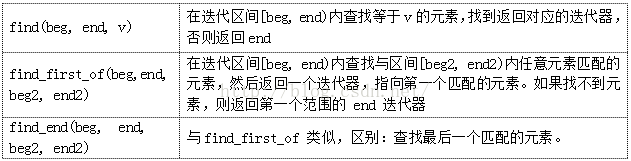find_if（beg，end，func）：函数find的带一个函数参数的_if版本，与find功能相同，条件：使函数func返回true。

搜索与统计算法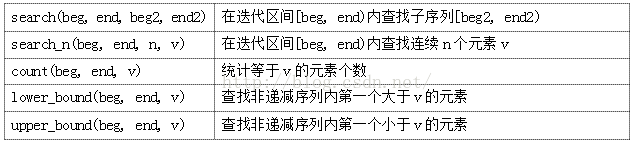count_if（beg，end，func）：函数count的_if版本。

#include <vector>
#include <algorithm>
#include <iostream>

using namespace std;

bool evenNum( int n ) //是否为偶数

{ return n % 2; }

void main()

{

int num = 6;

vector<int> v1;

for( int i = 0; i != 10; ++i )

v1.push_back(i);

vector<int>::iterator iter = find( v1.begin(), v1.end(), num ); //查找等于6的元素位置

if( iter != v1.end() )

cout << "匹配元素的索引: " << iter - v1.begin() << endl; //找到匹配元素位置6

vector<int> v2;

v2.push_back(6);

v2.push_back(5);

v2.push_back(3);

iter = find_first_of( v1.begin(), v1.end(), v2.begin(), v2.end() ); //第一个匹配元素是3

if( iter != v1.end() )

cout << "第一个匹配元素索引：" << iter - v1.begin() << endl;

int even_times = count_if( v1.begin(), v1.end(), evenNum ); //谓词函数参数，偶数个数为5个

cout << "偶数个数 ：" << even_times << endl;

vector<int> v3;

v3.push_back(1);

v3.push_back(1);

v3.push_back(2);

v3.push_back(2);

//v3 非递减，每次循环 iter 跳向第一个大于当前元素的位置，因此只输出两个数 1，2

for( iter = v3.begin(); iter != v3.end(); iter = lower_bound( iter, v3.end(), *iter ) )

cout << *iter << " ";

}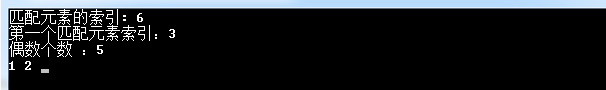二、可变序列算法

可变序列算法包括元素复制，变换，替换，填充，移除和随机生成等。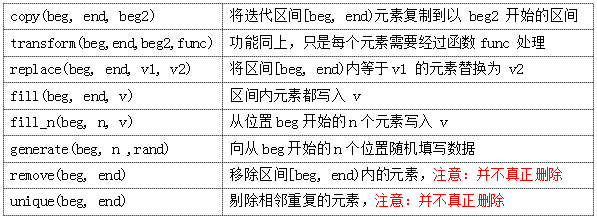copy，transform，fill_n和generat都需要保证：输出序列有足够的空间。

删除函数并不真正删除元素，只是将要删除的元素移动到容器的末尾，删除元素需要容器擦除函数来操作。同理，独特的函数也不会改变容器的大小，只是这些元素的顺序改变了，是将无重复的元素复制到序列的前端，从而覆盖相邻的重复元素.unique返回的迭代器指向超出无重复的元素范围末端的下一位置。

remove_if（beg，end，func）：remove的_if版本。

replace_if（beg，end，func，v2）：replace的_if版本。

_copy版本，需注意：必须保证输出序列的大小不小于输入序列的大小。

remove_copy（beg，end，dest）：remove的_copy版本，将反转后的序列输出到从dest开始的区间。

remove_copy_if（beg，end，dest，func）：remove_copy的_if版本。

replace_copy（beg，end，dest，v1，v2）：replace的_copy版本。

replace_copy_if（beg，end，dest，func，v2）：replace_copy的_if版本。

三、排序算法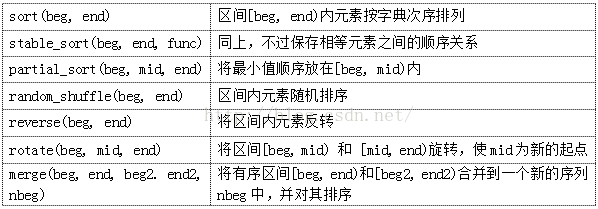partial_sort对区间[beg，end]内的mid - beg个元素进行排序，将最小的mid - beg个元素有序放在序列的前mid - beg的位置上。

reverse_copy（beg，end，dest）：reverse的_copy版本。

rotate_copy（beg，mid，end，dest）：rotate的_copy版本。

class A
{
public:
bool operator()(int i, int j)
{
return i > j;
}
};
int main()
{
vector<int> vec{ 12, 2, 6, 1, 8 };
A a;
sort(vec.begin(), vec.end(), a);

for (const auto& it : vec)
{
cout << it << endl;
}
return 0;
}

四、关系算法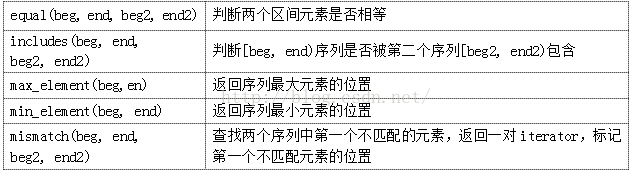标准库还提供求最大值，最小值的max和min函数。

五，堆算法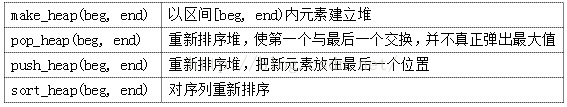vector<int> v;

v.push_back(3);

v.push_back(9);

v.push_back(17);

v.push_back(20);

v.push_back(12);

make_heap(v.begin(),v.end()); //用 vector 代替数组建立最大堆

cout << "堆： ";

for( vector<int>::iterator iter= v.begin(); iter != v.end(); ++iter )

cout << *iter << " ";

cout<<endl;

cout << "堆排序后： ";

sort_heap(v.begin(),v.end()); //堆排序

for( vector<int>::iterator iter = v.begin(); iter != v.end(); ++iter )

cout << *iter << " ";

cout<<endl;五、容器特有的算法

list容器上的迭代器是双向的，而不是随机访问类型。因此，在此容器上不能使用需要随机访问迭代器的算法。这些算法包括sort及其相关的算法。还有一些其他的泛型算法，如合并，删除，反向和唯一，虽然可以用在列上，但却付出了性能上的代价。如果这些算法利用列表容器实现的特点，则可以更高效地执行。

标准库为list容器定义了更精细的操作集合，使它不必只依赖于泛型操作。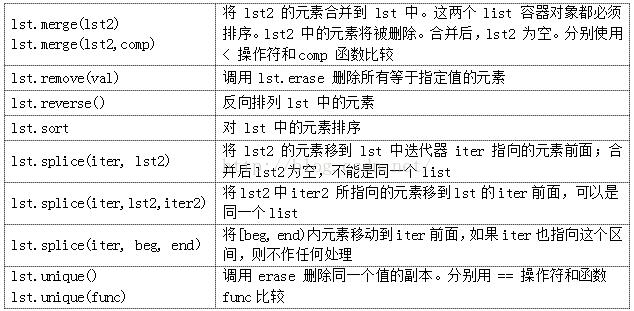lst.remove_if（func）：remove（）的_if版本，删除使用func返回真的元素。

展开全文weixin_38739598 2021-01-08 21:41:09
• 禁忌搜索算法 算法 机器学习

禁忌搜索算法(Tabu Search or Taboo Search,TS)是一种迭代搜索算法，靠记忆来引导算法的搜索过程。 1 算法原理 主要包含2个方面：局部领域搜索、禁忌搜索，在领域搜索的基础上，通过禁忌准则来避免重复搜索，通过...

禁忌搜索算法(Tabu Search or Taboo Search,TS)是一种迭代搜索算法，靠记忆来引导算法的搜索过程。

1 算法原理

主要包含2个方面：局部领域搜索、禁忌搜索，在领域搜索的基础上，通过禁忌准则来避免重复搜索，通过藐视准则来赦免一些被禁忌的优良状态，以实现全局优化。

1.1 局部领域搜索

局部领域搜索是基于贪婪准则持续在当前的领域中进行搜索，找到局部最优解。大致方法是选定一个可行解，并产生领域解集,逐一比较的目标值，选出最优解更新，直到找不到更优为止，记为

1.2 禁忌搜索

在局部搜索的基础上，建立禁忌表，记录近几次迭代过程中的移动（所谓移动，即产生领域解的变化），凡是处于禁忌表中的移动是不允许的，来避免算法重复访问最近几次已经访问过的解，从而防止陷入局部最优解。注意禁忌表记录可能是解，也可以是产生领域解的移动或变化。

1.3 藐视准则

藐视准则是为了不错过最优解。

一般来说，通过设置存在时间或禁忌表长度来实现特赦以前出现过的最优解相关移动。

2 算法流程

在此之前需要对解进行区分，当前解，领域解（领域中最优的解），候选解，全局最优解（也叫bestsofar）。以旅行商问题为例，当经过移动（随机交换2个城市的访问顺序）会产生好，就会升级成

步骤1   生成初始解和置禁忌表tabu为空

步骤2    从当前解产生若干领域解，并确定若干候选解

步骤3    如果优于，则更新=和将对应的禁忌对象加入禁忌表tabu，否则基于禁忌表tabu从中选出次优解，更新和tabu

步骤4    更新=和基于藐视准则更新tabu

步骤5    判断结束或继续步骤2-5

3 关键参数

领域结构

从一个解通过“移动”产生另一个解的途径，常用方法互换、插值、逆序

禁忌对象

即放入禁忌表中的变化元素，可以是状态本身（解本身）或状态分量（领域结构中的移动

候选解选择

切记要选择充分，保证在领域内充分搜索

一般是先进先出的队列

禁忌长度

禁忌对象在禁忌表中的任期，可以是常量也可以是变量

4 仿真实例

旅行商问题（TSP问题）

假设1个旅行商要对31个省会城市进行拜访，要求距离最短，不能重复拜访，且最终要回到出发城市。

31个城市坐标：

[1304 2312;3639 1315;4177 2244;3712 1399;3488 1535;3326 1556;
3238 1229;4196 1044;4312 790  ;4386 570  ;3007 1970;2562 1756;
2788 1491;2381 1676;1332 695  ;3715 1678;3918 2179;4061 2370;
3780 2212;3676 2578;4029 2838;4263 2931;3429 1908;3507 2376;
3394 2643;3439 3201;2935 3240;3140 3550;2545 2357;2778 2826;
2370 2975]；

代码

close all;
clear all;
clc;
%%%%%初始化参数
C = [1304 2312;3639 1315;4177 2244;3712 1399;3488 1535;3326 1556;
3238 1229;4196 1044;4312 790 ;4386 570 ;3007 1970;2562 1756;
2788 1491;2381 1676;1332 695 ;3715 1678;3918 2179;4061 2370;
3780 2212;3676 2578;4029 2838;4263 2931;3429 1908;3507 2376;
3394 2643;3439 3201;2935 3240;3140 3550;2545 2357;2778 2826;
2370 2975];
n=size(C,1);
D=zeros(n);
for i=1:n
for j=1:n
D(i,j)=((C(i,1)-C(j,1))^2 + (C(i,2)-C(j,2))^2)^0.5;
end
end
tabu=zeros(n);
tabuL=21;
Ca=200;
canum=zeros(Ca,n);
s0=randperm(n);
bestsofar=s0;
bestlen=func1(D,bestsofar,n);
g=1;
G=1000;
%%%%%算法循环
while g<G
%%%%%产生领域解canum,产生200个，只取前100
%%%%%确保交换不重复
A=zeros(Ca,2);
p=1;
while p<=Ca
A(p,:)=ceil(rand(1,2)*n);
while A(p,1)==A(p,2)
A(p,:)=ceil(rand(1,2)*n);
end
temp1=min(A(p,1),A(p,2));
A(p,2)=max(A(p,1),A(p,2));
A(p,1)=temp1;
if p==1
k=0;%查重
else
for j=1:p-1
if A(p,1)==A(j,1) && A(p,2)==A(j,2)
k=1;
break;
else
k=0;
end
end
end
if k==0
p=p+1;
end
end
for i=1:Ca
canum(i,:)=s0;
canum(i,[A(i,1),A(i,2)])=s0([A(i,2),A(i,1)]);
canumlen(i)=func1(D,canum(i,:),n);
end
[canumlen,index]=sort(canumlen);
canum=canum(index,:);
A=A(index,:);
bestca=canum(1:Ca/2,:);
%%%%%藐视准则
if canumlen(1) < bestlen    %领域解更优
bestlen=canumlen(1);
s0=bestca(1,:);
bestsofar=s0;
for i=1:n
for j=1:n
if tabu(i,j)~=0
tabu(i,j)=tabu(i,j)-1;
end
end
end
tabu(A(1,1),A(1,2))=tabuL;
else
for k=1:Ca/2
if tabu(A(k,1),A(k,2))==0
s0=bestca(k,:);
for i=1:n
for j=1:n
if tabu(i,j)~=0
tabu(i,j)=tabu(i,j)-1;
end
end
end
tabu(A(k,1),A(k,2))=tabuL;
break;
end
end
end
arrbestlen(g)=bestlen;
g=g+1;
end
%%%%%画图
for i=1:n-1
plot([C(bestsofar(i),1),C(bestsofar(i+1),1)],[C(bestsofar(i),2),C(bestsofar(i+1),2)],'o-');
hold on;
end
plot([C(bestsofar(n),1),C(bestsofar(1),1)],[C(bestsofar(n),2),C(bestsofar(1),2)],'ro-');
title([num2str(g),'次最短距离：',num2str(bestlen)]);
hold off;
%%%%%输出优化结果
figure;
plot(arrbestlen);
xlabel('迭代次数');
ylabel('目标函数值');
title('适应度进化曲线');
%%%%%路径长度函数
function result = func1(D,f,N)
len=D(f(1),f(N));
for j=2:N
len=D(f(j),f(j-1))+len;
end
result=len;
end

结果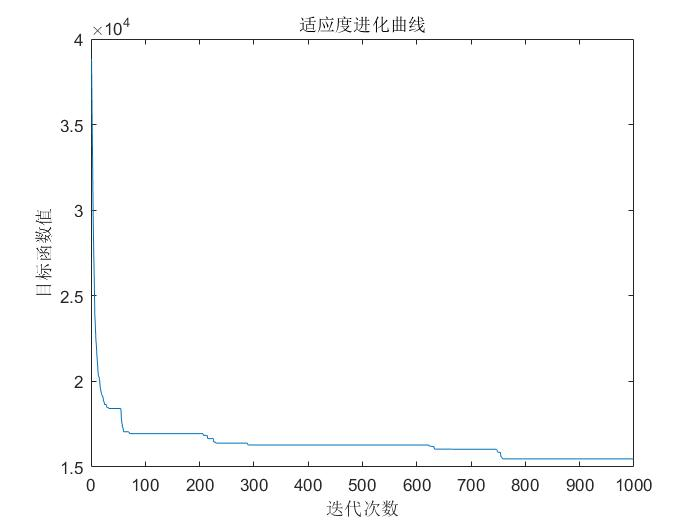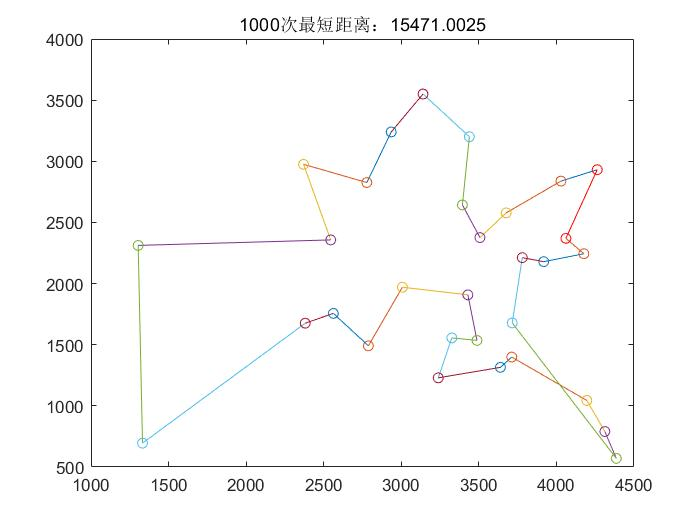展开全文talkAC 2021-11-20 14:32:29
• 常用算法框架 算法 数据结构 链表

qqzhaojianbiao 2021-11-10 14:21:58
• sanmi8276 2021-03-12 10:23:43
• 10分钟彻底理解自适应大邻域搜索算法 算法 自动驾驶 动态规划

qq_38361726 2021-10-11 10:13:47
• weixin_35057726 2021-07-10 02:36:17
• qq_47605698 2021-03-15 14:23:43
• 查找算法-4种常用的查找算法 算法 数据结构 java

GGB__ 2021-09-13 11:39:06
• 机器学习-常用回归算法归纳(全网之最) 机器学习 回归 人工智能

qq_42363032 2021-10-28 17:31:55
• 机器学习中的搜索算法 python 机器学习

newshandnews 2021-06-14 15:49:30
• 路径规划算法1.2图搜索算法——广度优先搜索、Dijkstra与A*寻路算法 算法 自动驾驶 自然语言处理

qq_41035283 2021-10-11 21:35:58
• weixin_39574868 2021-07-15 04:07:29
• 算法分析与设计中的五大常用算法 算法

weixin_43520670 2021-05-27 23:12:51
• weixin_29748637 2021-02-25 19:51:21
• weixin_33295562 2021-07-27 08:31:41
• 最短路径相关常用算法详解 算法 c++

weixin_46903770 2021-08-23 18:45:20
• Q1368089323 2021-01-03 23:26:16
• weixin_42539973 2020-12-31 03:41:55
• 常用算法模板总结--搜索与图论 算法 Java 数据结构

qq_40722827 2021-04-27 14:30:33
• 常见搜索算法（一）：深度优先和广度优先搜索 算法 DFS BFS

u010698107 2021-05-30 17:41:13
• 程序员常用十大算法（上） 算法 数据结构

a1489540461 2021-09-24 11:12:23
• 禁忌搜索算法学习笔记 python 深度学习 人工智能

liuqihang11 2021-07-13 20:31:42
• A* 路径搜索算法介绍及完整代码 python 算法 启发式算法

Zhefan_Xu 2021-08-05 15:09:40
• weixin_39962758 2021-02-28 18:54:18
• 路径规划算法1.1图搜索算法——可视图法 算法

qq_41035283 2021-09-14 20:37:18
• weixin_44364444 2021-01-26 21:04:35
• 最常用的五种算法设计思想 算法设计思想 字符串匹配算法 动态规划 贪心算法 分治法

CSDN2497242041 2021-04-06 23:42:02
• weixin_35698059 2021-03-09 17:22:42...